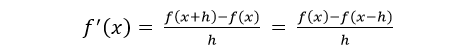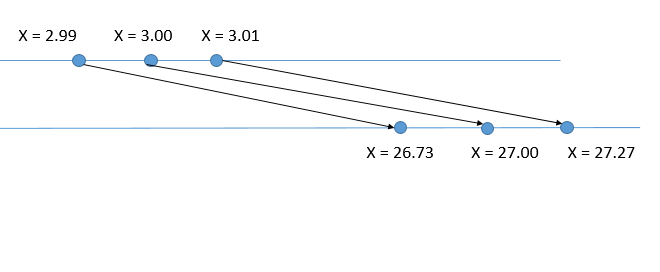Search
•Anand Zambare

# Derivatives: The physical significanceCalculus meme

Not to worry! we are going to discuss the physical sense of the derivatives not much of calculus.

In the famous Principia i.e. "Mathematical Principles of Natural philosophy." Newton put his thoughts about calculus as the science of physical nature (geometry) and its description through the generation of motion and its magnitude. Well, it was Leibnitz who was looking at the Tangent problems and explained calculus as the metaphysical explanation of the change in something w.r.t something.

Introduction :

Calculus and its applications are always the points of discussion from +2. The most important part of understanding the concepts in mathematics is the understanding of them in the real physical sense. As we start our journey in mathematics early in +2 it is a little easier to understand as we deal with a one-dimensional world or a two-dimensional world in a few cases. As we go ahead in say the BS or MS of our respective fields we start dealing with the 3D real world and then the understanding and extension of such concepts to that (3-D world) gets very much difficult. In this blog today, we are going to look at the derivatives and their actual physical significance in a few steps with extension to the three-dimensional world. There will be different levels of explanation for derivatives as we go along the article.

(Note: - Discussion is highly inclined towards the physical sense nothing about formulae is discussed here).

Derivatives as slope:

At the +2 level when we have first introduced the derivatives the most fundamental definition was given to us on that day itself! Let’s recall it before going ahead.Derivative from first principle

Here the ‘h’ is the distance between the two points i.e. ‘x+h’ and ‘x’ or ‘x’ and ‘x-h’. We recommend this as the most fundamental definition as we implement it when it comes to the CFD codes on computers. Anyway, going ahead for the physical significance the slope of a curve at a point is the most used definition for the derivative at +2. Here the curve is f(x) which is nothing but the y-axis and x is the x-axis on the graph. Look at the following figure to understand that.Derivative as a slope

As we can see, we start with X = a. Now the black line shown at X = a is the slope of the curve at X = a. This can be defined as the tangent of an angle made by line with respect to the horizontal axis (in this case it is the X-axis). This is given as the derivative of f(x) at x = a. Suppose we have some equation for curve f(x) then we can find its derivative and plug x = a, in the equation of f’(x) and get the slope value. This is the first level explanation which you all might be familiar with and if not then we hope now it’s crystal clear to you. Let’s go ahead and get deep insights into this definition.

Introduction to Linear Maps:

Before considering the next level explanation let’s look at what are the linear maps. Linear maps are nothing but the mapping of the given geometry into the new geometric domain. So essentially multiplication can be visualized as the stretching linear map. Consider an example where originally we have ‘x’ as a function. What this function does is it takes the input and without doing anything on it, it shall give us ‘x’ as it is our output. So if 100 is my input my output is also going to be 100. But if f(x) says ‘5x’ then for an input of 100 my output is going to be 500. Meaning we have stretched it 5 times. This can be considered as a linear map. The general form of one linear map can be considered as ‘ax+b’ where ‘a’ is the stretching factor and ‘b’ is translating factor. Following graphical illustration may help in visualizing the linear maps.Linear maps

As we can see the black axis is the original geometrical domain and the dotted blue lines are the linearly mapped blue domain. The origin which is orange will be having the same coordinates irrespective of the original or mapped axes. The coordinates of the two points (0, 1) and (1, 0) are now different in the mapped geometry. For now consider them as (x1, y1) and (x2, y2) respectively. We can consider the matrix from using this (x2, y2) and (x1, y1), and that matrix is called as ‘Jacobian’ of the mapping which is written as follows.Jacobian

This matrix can be further used to find the coordinates of all required points just by multiplication. This idea is sufficient for understanding the derivatives in multidimensional form. We will look at more details of the linear mappings and Jacobians in the upcoming blogs of mathematics in depth.

Derivatives as linear maps:

Now let’s look at the derivatives as the linear transformations. Consider a function f(x) which is say x cube. Now take the original number line as an input to this function, we will consider only the positive side for convenience. The function f(x) maps all the inputs as x cube. Meaning x = 1 is mapped as 1, 2 is mapped on 8, 3 on 27, and so on. Now take the derivative of the f(x) which is 3 times the x squared.Let’s examine the derivative around ‘2’. The value of the derivative at x =2 is 12. We can see that the point 1.99 is mapped on the 7.880, 2 is mapped on 8 and 2.01 is mapped on the 8.120, now from this, we can see clearly that the f’(x) value is 12 and 0.12 is the scale of the dilation around the numbers we are observing. Consider 3, 2.99, and 3.01 as the new inputs, the value of the slope is 27 and the dilation factor should be 0.27. 2.99 is mapped to 26.73, 3 is mapped to 27 and 3.01 is mapped to 27.27 so we can say the slope by 100 is the dilation factor approximately. Since 2 and 3 are very small values this approximation is kind of good. Now, take x = 20, 19.99 and 20.01. 20 is mapped on 8000. 19.99 is mapped on 7988.006 and x = 20.01 is mapped on 8012.006 the slope value is 1200 and the slope/100 is 12 this logic of slope being the dilation factor still looks valid. Following number lines may make it clear.Linear map of derivatives near x = 2.Linear map of derivatives at x = 3.Linear map of derivatives at x = 20

So now we can definitely say that slope behaves like some sort of linear map around the ‘x’ value approximately in one dimension. What about two dimensions? Let’s find it. In two dimensions the derivatives are partial derivatives. Meaning if we have a function of x as well as y then the derivative is always w.r.t either x or y. So, what does it mean physically? Actually, this is just an extension of the ‘Derivatives as linear maps’. Look at the procedure of finding the derivatives. If we are finding the derivative with respect to x then we keep y as constant and vice-a-versa. So we can say that derivative w.r.t x is a linear map that treats the variable ‘y’ as a constant and maps the geometry as we have seen earlier. This is the logic that can be extended to 3-D functions.

So overall looking at the physical significance and the most generalized definition which is extendable to any dimension appears to be the ‘approximated linear maps for every specific input’.

Linear maps can have the following properties:

1) Parallel lines stay parallel.

2) Even spacings are preserved.

3) Origin is fixed.

With this, we will end this article and continue looking at the essence of calculus as we go along in the series of articles in mathematics.

Summary:

• Derivatives represent the slope for basic 1D cases. In general, it can be said it’s the rate of change of dependent variable w.r.t independent variable.

• Derivatives can be generalized as a linear map. This is an extendable definition for 2D and 3D geometry also.

• Jacobian of a linear map can be used to find each and every point on the regular domain on the transformed domain.